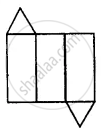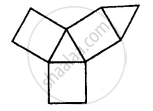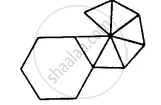# Name the Polyhedron that Can Be Made by Folding Each of the Following Nets: - Mathematics

Short Note

Name the polyhedron that can be made by folding each of the following nets:

(i)(ii)(iii)#### Solution

(i) Triangular prism. It has 3 rectangles and 2 triangles.
(ii) Triangular prism. It has 3 rectangles and 2 triangles.
(iii) Hexagonal pyramid as it has a hexagonal base and 6 triangles.

Is there an error in this question or solution?
Chapter 19: Representing 3-D in 2-D - Exercise 19 [Page 220]

#### APPEARS IN

Selina Concise Mathematics Class 8 ICSE
Chapter 19 Representing 3-D in 2-D
Exercise 19 | Q 12 | Page 220

Share